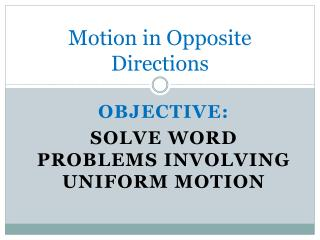DownloadDownload PresentationMotion in Opposite Directions

# Motion in Opposite Directions

Télécharger la présentation## Motion in Opposite Directions

- - - - - - - - - - - - - - - - - - - - - - - - - - - E N D - - - - - - - - - - - - - - - - - - - - - - - - - - -
##### Presentation Transcript

1. Motion in Opposite Directions Objective: Solve word problems involving uniform motion

2. Uniform Motion • Moving at a speed that isnot changing.

3. Distance = Rate x Time D = RT (dirt) Rate x Time = Distance 50mph x 2hours = 100miles

4. D/T = R Distance / Time = Rate 100miles / 2hours = 50mph

5. Distance = Rate x Time D/R = T Distance /Rate = Time 100miles / 50mph = 2hours

6. Solving Word Problems • 1. Draw a sketch • 2. Identify Unknowns • 3. Assign Variable • 4. Make a Chart • 5. Write and solve an equation

7. Equation for Motion in Opposite Directions Add distance for both objects and setequal to the total distance apart.

8. Example • Bike riders Brent and Jane started at noon from points 60 km apart and rode toward each other, meeting at 1:30pm. Brent’s speed was 4km/h greater than Jane’s speed. • Find their speeds.

9. Start riding at 12pm & meet at 1:30pm r r+4 60 km

10. Unknown Jane and Brent’s speed.

11. Variables • Let r = Jane’s rate • Let r + 4 = Brent rate • Make a chart and use the information to label your diagram

12. Chart x = r 1.5 1.5r r + 4 1.5 1.5(r + 4)

13. Equation • 1.5 ( r + 4) + 1.5r = 60 • r = 18 Jane’s speed • r + 4 = 22 Brent’s speed Brent’s speed was 22km/h and Jane’s speed was 18 km/h

14. Try This • At noona private plane left Austin for Los Angeles, 2100km away, flying at 500 km/h. One hour later a jet left Los Angeles for Austin at700km/h. At what time did they pass each other?

15. 700 km 2100 km 500 km

16. 500t + 700(t – 1) = 2100

17. 500t + 700(t – 1) = 2100 500t +700t – 700 = 2100 1200t – 700 = 2100 +700 =+700 1200t = 2800 1200t/1200 =2800/1200 T=2 and 400/1200 HRS or 2 and 1/3hrs is 2hrs 20min WHATS THE QUESTION Airplane took 2 hours and 20 min before meeting with Jet that took t – 1 =2hr 20 min – 1 = 1hr and 20 min Time is 12:00 + 2 hr and 20 min . They jet passed the plane at 2:20